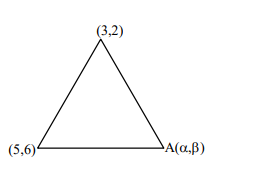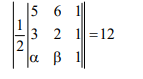# Let A be the set of all points`
Question:

Let $A$ be the set of all points $(\alpha, \beta)$ such that the area of triangle formed by the points $(5,6),(3,2)$ and $(\alpha, \beta)$ is 12 square units. Then the least possible length of a line segment joining the origin to a point in A, is:

1. $\frac{4}{\sqrt{5}}$

2.  $\frac{16}{\sqrt{5}}$

3. $\frac{8}{\sqrt{5}}$

4. $\frac{12}{\sqrt{5}}$

Correct Option: , 3

Solution:$4 \alpha-2 \beta=\pm 24+8$

$\Rightarrow 4 \alpha-2 \beta=+24+8 \Rightarrow 2 \alpha-\beta=16$

$2 x-y-16=0$ $\ldots(1)$

$\Rightarrow 4 \alpha-2 \beta=-24+8 \Rightarrow 2 \alpha-\beta=-8$

$2 x-y+8=0$ ........(2)

perpendicular distance of (1) from $(0,0)$

$\left|\frac{0-0-16}{\sqrt{5}}\right|=\frac{16}{\sqrt{5}}$

perpendicular distance of $(2)$ from $(0,0)$ is

$\left|\frac{0-0+8}{\sqrt{5}}\right|=\frac{8}{\sqrt{5}}$